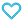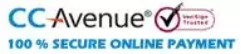# Rapid Mathematics-Crash Course for Peak Performance

225.00

Discount offer on this book in a bundle, click to view

Rapid Mathematics is a high concept book for all the CBSE and ICSE students to take a fast and easy learning and having maths formula on their fingertips. It helps them memorise and relearn all the mathematics formula and concepts. It contains the complete syllabus of NCERT books of Class XI and XII. It is designed by our MTG experts as per the need of CBSE and ICSE students preparing for all the competitive exams of Engineering. Rapid Mathematics is a high yield facts book for your fast and rapid revision of course NCERT books.

953 in stock

Qty:Add to Wishlist### TABLE OF CONTENT:

• Sets, Relations, Functions and Binary Operations
• Complex Numbers
• Sequences and Series
• Permutations and Combinations
• Binomial Theorem
• Exponential and Logarithmic Series
• Matrices
• Determinants
• Cartesian System of Rectangular Co-ordinates and Straight Lines
• Family of Lines
• Circles
• Parabola
• Ellipse
• Hyperbola
• Real Functions
• Limits
• Continuity and Differentiability
• Differentiation
• Tangents, Normals and Other Applications of Derivatives
• Increasing and Decreasing Functions
• Maxima and Minima
• Indefinite Integrals
• Definite Integrals
• Areas of Bounded Regions
• Differential Equations
• Vectors
• Three Dimensional Geometry
• Probability
• Trigonometry
• Statistics
• Linear Programming
• Partial Fraction
• Mathematical Reasoning
ISBN11 9789389971125 MTG Editorial Board 2020-21 200 Class 11, Class 12 Regional Ent. Exams-Engg Mathematics 231gm

## Reviews

There are no reviews yet.